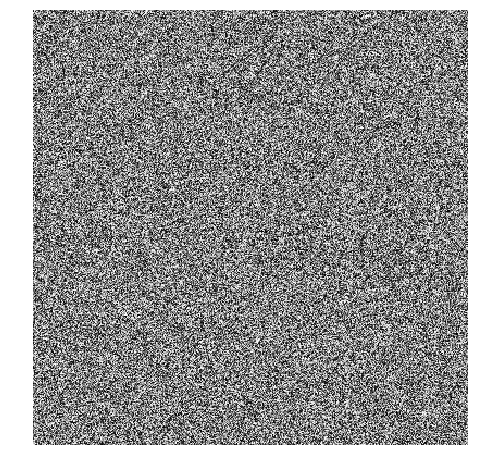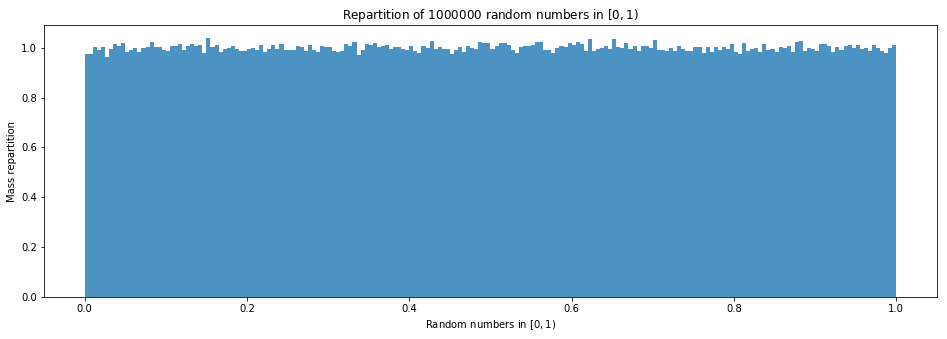# Manual implementation of the Mersenne twister PseudoRandom Number Generator (PRNG)¶

This small notebook is a short experiment, to see if I can implement the Mersenne twister PseudoRandom Number Generator (PRNG).

And then I want to use it to define a rand() function, and use it to samples from the most famous discrete and continuous probability distributions. Random permutations will also be studied.

## Common API for the PRNG defined here¶

First, I want to define a simple object-oriented API, in order to write all the examples of PNRG with the same interface.

In :
import numpy as np

In :
class PRNG(object):
"""Base class for any Pseudo-Random Number Generator."""
def __init__(self, X0=0):
"""Create a new PRNG with seed X0."""
self.X0 = X0
self.X = X0
self.t = 0
self.max = 0

def __iter__(self):
return self

def seed(self, X0=None):
"""Reinitialize the current value with X0, or self.X0.

- Tip: Manually set the seed if you need reproducibility in your results.
"""
self.t = 0
self.X = self.X0 if X0 is None else X0

def __next__(self):
"""Produce a next value and return it."""
# This default PRNG does not produce random numbers!
self.t += 1
return self.X

def randint(self, *args, **kwargs):
"""Return an integer number in [| 0, self.max - 1 |] from the PRNG."""
return self.__next__()

def int_samples(self, shape=(1,)):
"""Get a numpy array, filled with integer samples from the PRNG, of shape = shape."""
# return [ self.randint() for _ in range(size) ]
return np.fromfunction(np.vectorize(self.randint), shape=shape, dtype=int)

def rand(self, *args, **kwargs):
"""Return a float number in [0, 1) from the PRNG."""
return self.randint() / float(1 + self.max)

def float_samples(self, shape=(1,)):
"""Get a numpy array, filled with float samples from the PRNG, of shape = shape."""
# return [ self.rand() for _ in range(size) ]
return np.fromfunction(np.vectorize(self.rand), shape=shape, dtype=int)


## First example: a simple linear congruential generator¶

Let me start by implementing a simple linear congruential generator, with three parameters $m$, $a$, $c$, defined like this :

• Start from $X_0$,
• And then follow the recurrence equation: $$X_{t+1} = (a X_t + c) \mod m.$$

This algorithm produces a sequence $(X_t)_{t\in\mathbb{N}} \in \mathbb{N}^{\mathbb{N}}$.

In :
class LinearCongruentialGenerator(PRNG):
"""A simple linear congruential Pseudo-Random Number Generator."""
def __init__(self, m, a, c, X0=0):
"""Create a new PRNG with seed X0."""
super(LinearCongruentialGenerator, self).__init__(X0=X0)
self.m = self.max = m
self.a = a
self.c = c

def __next__(self):
"""Produce a next value and return it, following the recurrence equation: X_{t+1} = (a X_t + c) mod m."""
self.t += 1
x = self.X
self.X = (self.a * self.X + self.c) % self.m
return x


The values recommended by the authors, Lewis, Goodman and Miller, are the following:

In :
m = 1 << 31 - 1  # 1 << 31 = 2**31
a = 7 ** 4
c = 0


The seed is important. If $X_0 = 0$, this first example PRNG will only produce $X_t = 0, \forall t$.

In :
FirstExample = LinearCongruentialGenerator(m=m, a=a, c=c)

In :
def test(example, nb=3):
for t, x in enumerate(example):
print("{:>3}th value for {.__class__.__name__} is X_t = {:>10}".format(t, example, x))
if t >= nb - 1:
break

In :
test(FirstExample)

  0th value for LinearCongruentialGenerator is X_t =          0
1th value for LinearCongruentialGenerator is X_t =          0
2th value for LinearCongruentialGenerator is X_t =          0


But with any positive seed, the sequence will appear random.

In :
SecondExample = LinearCongruentialGenerator(m=m, a=a, c=c, X0=12011993)

In :
test(SecondExample)

  0th value for LinearCongruentialGenerator is X_t =   12011993
1th value for LinearCongruentialGenerator is X_t =  923507769
2th value for LinearCongruentialGenerator is X_t =   65286809


The sequence is completely determined by the seed $X_0$:

In :
SecondExample.seed(12011993)
test(SecondExample)

  0th value for LinearCongruentialGenerator is X_t =   12011993
1th value for LinearCongruentialGenerator is X_t =  923507769
2th value for LinearCongruentialGenerator is X_t =   65286809


Note: I prefer to use this custom class to define iterators, instead of a simple generator (with yield keyword) as I want them to have a .seed(X0) method.

## Trying to write a cell in cython, for speeding things up¶

For more details, see this blog post, and this other one.

In :
# Thanks to https://nbviewer.jupyter.org/gist/minrk/7715212
from __future__ import print_function
from IPython.core import page
def myprint(s):
try:
print(s['text/plain'])
except (KeyError, TypeError):
print(s)
page.page = myprint

In :
%load_ext cython

The cython extension is already loaded. To reload it, use:


Then we define a function LinearCongruentialGenerator_next, in a Cython cell.

In :
%%cython
def nextLCG(int x, int a, int c, int m):
"""Compute x, nextx = (a * x + c) % m, x in Cython."""
cdef int nextx = (a * x + c) % m
return (x, nextx)

In :
from __main__ import nextLCG
nextLCG
nextLCG?

Out:
<function _cython_magic_dde6682b939b6e9ea0a22da681a4bea1.nextLCG>
Docstring: Compute x, nextx = (a * x + c) % m, x in Cython.
Type:      builtin_function_or_method



Then it's easy to use it to define another Linear Congruential Generator.

In :
class CythonLinearCongruentialGenerator(LinearCongruentialGenerator):
"""A simple linear congruential Pseudo-Random Number Generator, with Cython accelerated function __next__."""

def __next__(self):
"""Produce a next value and return it, following the recurrence equation: X_{t+1} = (a X_t + c) mod m."""
self.t += 1
x, self.X = nextLCG(self.X, self.a, self.c, self.m)
return x


Let compare it with the first implementation (using pure Python).

In :
NotCythonSecondExample = LinearCongruentialGenerator(m=m, a=a, c=c, X0=13032017)
CythonSecondExample = CythonLinearCongruentialGenerator(m=m, a=a, c=c, X0=13032017)


They both give the same values, that's a relief.

In :
test(NotCythonSecondExample)
test(CythonSecondExample)

  0th value for LinearCongruentialGenerator is X_t =   13032017
1th value for LinearCongruentialGenerator is X_t =  151359921
2th value for LinearCongruentialGenerator is X_t =  490433809
0th value for CythonLinearCongruentialGenerator is X_t =   13032017
1th value for CythonLinearCongruentialGenerator is X_t =  151359921
2th value for CythonLinearCongruentialGenerator is X_t =  490433809


The speedup is not great, but still visible.

In :
%timeit [ NotCythonSecondExample.randint() for _ in range(1000000) ]
%timeit [ CythonSecondExample.randint() for _ in range(1000000) ]

1 loop, best of 3: 766 ms per loop
1 loop, best of 3: 729 ms per loop

In :
%prun min(CythonSecondExample.randint() for _ in range(1000000))

         4000005 function calls in 1.291 seconds

Ordered by: internal time

ncalls  tottime  percall  cumtime  percall filename:lineno(function)
1000000    0.481    0.000    0.584    0.000 <ipython-input-145-d36c39b118f6>:4(__next__)
1000001    0.372    0.000    1.184    0.000 <string>:1(<genexpr>)
1000000    0.228    0.000    0.812    0.000 <ipython-input-132-93560edf79a4>:28(randint)
1    0.107    0.107    1.291    1.291 {built-in method builtins.min}
1000000    0.103    0.000    0.103    0.000 {_cython_magic_dde6682b939b6e9ea0a22da681a4bea1.nextLCG}
1    0.000    0.000    1.291    1.291 {built-in method builtins.exec}
1    0.000    0.000    1.291    1.291 <string>:1(<module>)
1    0.000    0.000    0.000    0.000 {method 'disable' of '_lsprof.Profiler' objects}


## Checking and plotting the result?¶

First, we can generate a matrix of samples, as random floats in $[0, 1)$, and check that the mean is about $1/2$:

In :
shape = (400, 400)
image = SecondExample.float_samples(shape)

In :
np.mean(image), np.var(image)

Out:
(0.4996821506033815, 0.083393476821803994)

What about the speed? Of course, a hand-written Python code will always be really slower than a C-extension code, and the PRNG from the modules random or numpy.random are written in C (or Cython), and so will always be faster. But how much faster?

In :
import random
import numpy.random

print(np.mean(SecondExample.float_samples(shape)))
print(np.mean([ [ random.random() for _ in range(shape) ] for _ in range(shape) ]))
print(np.mean(numpy.random.random(shape)))

0.500181275654
0.500273447015
0.499487700229

In :
%timeit SecondExample.float_samples(shape)
%timeit [ [ random.random() for _ in range(shape) ] for _ in range(shape) ]
%timeit numpy.random.random(shape)

1 loop, best of 3: 271 ms per loop
100 loops, best of 3: 22.2 ms per loop
1000 loops, best of 3: 1.56 ms per loop


This was expected: of course numpy.random. functions are written and optimized to generate thousands of samples quickly, and of course my hand-written Python implementation for LinearCongruentialGenerator is slower than the C-code generating the module random.

We can also plot this image as a grayscaled image, in order to visualize this "randomness" we just created.

In :
%matplotlib inline
import matplotlib.pyplot as plt

def showimage(image):
plt.figure(figsize=(8, 8))
plt.imshow(image, cmap='gray', interpolation='none')
plt.axis('off')
plt.show()

In :
showimage(image)It looks good already! We can't see any recurrence, but we see a regularity, with small squares.

And it does not seem to depend too much on the seed:

In :
SecondExample.seed(11032017)
image = SecondExample.float_samples((10, 10))
showimage(image)In :
SecondExample.seed(1103201799)
image = SecondExample.float_samples((10, 10))
showimage(image)We can also visualize the generated numbers with a histogram, to visually check that the random numbers in $[0, 1)$ are indeed "uniformly" located.

In :
def plotHistogram(example, nb=100000, bins=200):
numbers = example.float_samples((nb,))
plt.figure(figsize=(16, 5))
plt.hist(numbers, bins=bins, normed=True, alpha=0.8)
plt.xlabel("Random numbers in $[0, 1)$")
plt.ylabel("Mass repartition")
plt.title("Repartition of ${}$ random numbers in $[0, 1)$".format(nb))
plt.show()

In :
plotHistogram(SecondExample, 1000000, 200)## A second example: Multiple-Recursive Generator¶

Let start by writing a generic Multiple Recursive Generator, which is defined by the following linear recurrence equation, of order $k \geq 1$:

• Start from $X_0$, with a false initial history of $(X_{-k+1}, X_{-k}, \dots, X_{-1})$,
• And then follow the recurrence equation: $$X_{t} = (a_1 X_{t-1} + \dots + a_k X_{t-k}) \mod m.$$

This algorithm produces a sequence $(X_t)_{t\in\mathbb{N}} \in \mathbb{N}^{\mathbb{N}}$.

In :
class MultipleRecursiveGenerator(PRNG):
"""A Multiple Recursive Pseudo-Random Number Generator (MRG), with one sequence (X_t)."""
def __init__(self, m, a, X0):
"""Create a new PRNG with seed X0."""
assert np.shape(a) == np.shape(X0), "Error: the weight vector a must have the same shape as X0."
super(MultipleRecursiveGenerator, self).__init__(X0=X0)
self.m = self.max = m
self.a = a

def __next__(self):
"""Produce a next value and return it, following the recurrence equation: X_t = (a_1 X_{t-1} + ... + a_k X_{t-k}) mod m."""
self.t += 1
x = self.X
nextx = (np.dot(self.a, self.X)) % self.m
self.X[1:] = self.X[:-1]
self.X = nextx
return x


For example, with an arbitrary choice of $k = 3$, of weights $a = [10, 9, 8]$ and $X_0 = [10, 20, 30]$:

In :
m = (1 << 31) - 1
X0 = np.array([10, 20, 30])
a = np.array([10, 9, 8])

ThirdExample = MultipleRecursiveGenerator(m, a, X0)

test(ThirdExample)

  0th value for MultipleRecursiveGenerator is X_t =         10
1th value for MultipleRecursiveGenerator is X_t =        520
2th value for MultipleRecursiveGenerator is X_t =       5450


We can again check for the mean and the variance of the generated sequence:

In :
shape = (400, 400)
image = ThirdExample.float_samples(shape)
np.mean(image), np.var(image)

Out:
(0.49952060992684566, 0.083438346428071117)

This Multiple Recursive Generator is of course slower than the simple Linear Recurrent Generator:

In :
%timeit SecondExample.float_samples(shape)
%timeit ThirdExample.float_samples(shape)

1 loop, best of 3: 221 ms per loop
1 loop, best of 3: 895 ms per loop


And it seems to work fine as well:

In :
showimage(image)